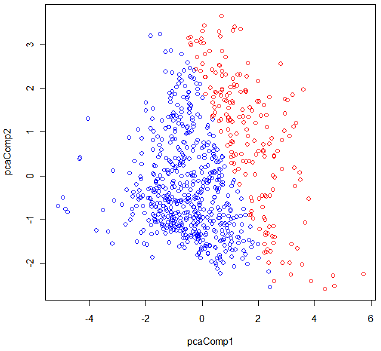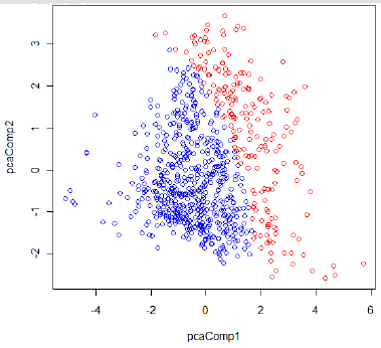# 9.2.10 - R ScriptsPrinter-friendly version

#### 1) Acquire Data

Diabetes data

The diabetes data set is taken from the UCI machine learning database repository at: https://archive.ics.uci.edu/ml/datasets/Pima+Indians+Diabetes.

• 768 samples in the dataset
• 8 quantitative variables
• 2 classes; with or without signs of diabetes

Load data into R as follows:

# set the working directory
setwd("C:/STAT 897D data mining")
# comma delimited data and no header for each variable

In RawData, the response variable is its last column; and the remaining columns are the predictor variables.

responseY <- as.matrix(RawData[,dim(RawData)])
predictorX <- as.matrix(RawData[,1:(dim(RawData)-1)])

For the convenience of visualization, we take the first two principle components as the new feature variables and conduct k-means only on these two dimensional data.

pca <- princomp(predictorX, cor=T) # principal components analysis using correlation matrix

predict(model.lda)$class The classification result by LDA is shown in Figure 1. The red circles correspond to Class 1 (with diabetes), the blue circles to Class 0 (non-diabetes).Figure 1: Classification result by LDA #### 3) Quadratic Discriminant Analysis Quadratic discriminant analysis does not assume homogeneity of the covariance matrices of all the class. The following code performs the QDA. model.qda <- qda(responseY ~ pc.comp1+pc.comp2) Again, we can use predict()$class to obtain classification result based on the estimated QDA model.

predict(model.qda)\$class

The classification result by QDA is shown in Figure 2.Figure 2: Classification result by QDA